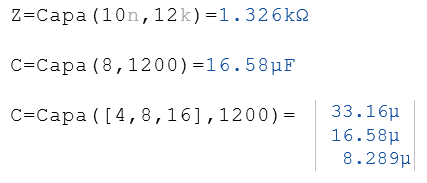# Capa Function

Calculates the capacitance, the impedance, or the frequency of a capacitor

## Description of the Capa function

The Capa function calculates either the capacitance, the frequency or the impedance for a capacitor. Two of the values must be passed as parameters to the function in order to calculate the third one. The order of the arguments is arbitrary. The parameters can be real numbers or lists of real numbers.

C = Capa (f, Z)

f = Capa (C, Z)

Z = Capa (f, C)

### Legend

 C Capacity in farads f Frequency in Hz Z Impedance in ohms

### Example